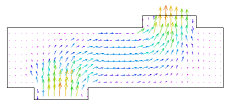﻿ Sample Problems > Applications > Fluids > 3d_flowbox

# 3d_flowbox

Navigation:  Sample Problems > Applications > Fluids >

# 3d_flowbox{  3D_FLOWBOX.PDE

This problem demonstrates the use of FlexPDE in 3D fluid flow.  It shows the flow of fluid

through a plenum box with a circular inlet at the bottom and an offset circular outlet at the top.

The corners of the duct are beveled to remove discontinuities in the flow, and the inlet pressure

is arbitrarily set at 0.05 units.

The solution uses a "penalty pressure", in which the pressure variable is used merely to guarantee

mass conservation. This technique is described in more detail in VISCOUS.PDE.

The order of interpolation is staged from linear to quadratic to cubic, which allows FlexPDE

to solve for the general distribution with far fewer unknowns, and then solve for more detail

in later stages.

Adaptive mesh refinement is turned off for speed in demonstration.

}

 title '3D flow through a plenum'   coordinates  cartesian3   variables  v(1e-6) = vector(vx,vy,vz)  p   select  order = staged(1,2,3)  regrid = off   definitions  long = 2  wide = 1  high = 1/2  xin = -1   yin = 0  xout = 1   yout = 0  rc = 0.5  duct = 0.2   { construct beveled surface }  b = 0.05 ! duct bevel  r2 = sqrt((x-xin)^2+(y-yin)^2)  H2 = -high-(rc+b)  z2 = max(min(H2+r2,-high),-high-b)  r3 = sqrt((x-xout)^2+(y-yout)^2)  H3 = high+(rc+b)  z3 = min(max(H3-r3,high),high+b)    dens = 1       { fluid density }  visc = 0.01     { fluid viscosity }  vm = magnitude(v)    div_v = dx(vx) + dy(vy) + dz(vz)    pfactor = 500  PENALTY = pfactor*visc/high^2    Pin = 0.05  Pout = 0   initial values  v = vector(0,0,0)p = Pin+(Pout-Pin)*(z+high+duct)/(2*high+2*duct)

equations

extrusion z = -high-duct,z2,z3,high+duct

boundaries

region 1   { plenum box }

surface 2 value(v) = vector(0,0,0) natural(p)=0

surface 3 value(v) = vector(0,0,0) natural(p)=0

layer 1 void

layer 3 void

start(-long,-wide)

value(v) = vector(0,0,0) natural(p)=0   { fix all side values }

line to (long,-wide)

to (long,wide)

to (-long,wide)

to close

limited region 2   { input hole }

layer 1

surface 1 natural(v) = vector(0,0,0) value(p)=Pin     { input duct opening }

start(xin,yin-rc)

layer 1   value(v) = vector(0,0,0) natural(p)=0 { duct sidewall drag }

surface 2 mesh_spacing=rc/2

arc(center=xin,yin) angle=360

limited region 3   { exit hole }

layer 3

surface 4 natural(v) = vector(0,0,0) value(p)=Pout   { output duct opening }

start(xout,yout-rc)

layer 3   value(v) = vector(0,0,0) natural(p)=0 { duct sidewall drag }

surface 3 mesh_spacing=rc/2

arc(center=xout,yout) angle=360

limited feature

surface 2

start(xin,yin-rc-b) arc(center=xin,yin) angle=360

limited feature

surface 3

start(xout,yout-rc-b) arc(center=xout,yout) angle=360

monitors

grid(x,z) on y=0

contour(vx) on x=0 report dens report pin

contour(vx) on y=0 report dens report pin

contour(vz) on y=0 report dens report pin

vector(vx,vz)on y=0 report dens report pin

contour(vx) on z=0 report dens report pin

contour(vy) on z=0 report dens report pin

contour(vz) on z=0 report dens report pin

vector(vx,vy)on z=0 report dens report pin

contour(p)   on y=0 report dens report pin

contour(div_v)   on y=0 report dens report pin

plots

grid(x,z) on y=0

contour(vx) on x=0 report dens report pin

contour(vx) on y=0 report dens report pin

contour(vz) on y=0 report dens report pin

vector(vx,vz)on y=0 report dens report pin

contour(vx) on z=0 report dens report pin

contour(vy) on z=0 report dens report pin

contour(vz) on z=0 report dens report pin

vector(vx,vy)on z=0 report dens report pin

contour(p)   on y=0 report dens report pin

contour(div_v)   on y=0 report dens report pin

summary

report(sintegral(vz,1,2)) as "Inflow"

report(sintegral(vz,4,3)) as "Outflow"

report(sintegral(vz,4,3)/sintegral(vz,1,2)) as "Ratio"

end